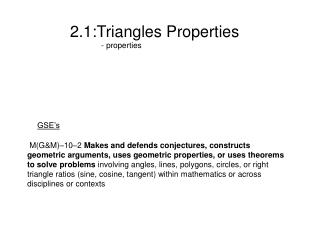Download Presentation2.1:Triangles Properties

# 2.1:Triangles Properties

Download Presentation## 2.1:Triangles Properties

- - - - - - - - - - - - - - - - - - - - - - - - - - - E N D - - - - - - - - - - - - - - - - - - - - - - - - - - -
##### Presentation Transcript

1. - properties 2.1:Triangles Properties GSE’s M(G&M)–10–2 Makes and defends conjectures, constructs geometric arguments, uses geometric properties, or uses theorems to solve problems involving angles, lines, polygons, circles, or right triangle ratios(sine, cosine, tangent) within mathematics or across disciplines or contexts

2. Triangles • Triangle-figure formed by 3 segments joining ______________ • Triangles are named by these three pts ______ • Would it matter if you named in a different order? R Q S

3. A C B Parts of a Triangle • Sides • Vertices • Angles

4. 2 Ways to classify triangles 1) by their Angles 2) by their Sides

5. 1)Angles • Acute- • Obtuse- • Right- • Equiangular-

6. 2) Sides • Scalene • Isosceles • Equilateral - No sides congruent -2 sides congruent - All sides are congruent

7. Converse of the Pythagorean Theorem Where c is chosen to be the longest of the three sides: If a2 + b2 = c2, then the triangle is ______. If a2 + b2 > c2 , then the triangle is _____. If a2 + b2 < c2, then the triangle is _____.

8. Example of the converse • Name the following triangles according to their angles 1) 4in , 8in, 9 in 2) 5 in , 12 in , 13 in 4) 10 in, 11in, 12 in

9. Example on the coordinate plane • Given DAR with vertices D(1,6) A (5,-4) R (-3, 0) Classify the triangle based on its sides and angles. Ans: DA = AR = DR =

10. Name the triangle by its angles and sides

11. Isosceles Triangle Vertex- A Leg Base Angles: C B Base-

12. Example Triangle TAP is isosceles with angle P as the Vertex. TP = 14x -5 , TA = 6x + 11 , PA = 10x + 43. Is this triangle also equilateral? P 14x-5 10x + 43 6x + 11 T A

13. 1. 2.

14. How can ΔABC be right and isosceles? A _____ ____ B C

15. Example • BCD is isosceles with BD as the base. Find the perimeter if BC = 12x-10, BD = x+5 CD = 8x+6 C 12x-10 8x+6 base D B X+5 Final answer

16. Example 2 Solve for x . Ans: (5x+24) + (5x+24) + (4x+6) = 180 5x +24 + 5x+ 24 + 4x+6 = 180 14x + 54 = 180 14x = 126 x = 9 5x +24

17. Exterior Angles Thm • The measure of an exterior angle of a triangle is equal to the sum of the measures of the 2 nonadjacent interior angles. • m1=mA+ mB m1= + A 1 B C

18. Example Find every angle measure Exterior angle = 2 nonadjacent angles 65 115 40

19. Exterior angle example Solve for q

20. Example Find the missing angles 80 80 60 40 50 50 70 110 110 70

21. Assignment